Start typing, then use the up and down arrows to select an option from the list.Jules Bruno
160views
3
6
Hey, guys, In this new video, we're gonna take a look at our Sala Bility product Constant, also called K SP. So here it says, find a sigh ability of cobalt three chloride, The soluble ity product constant or K sp that I give to you is 2.8 times 10 to the negative 13 and here we're gonna have to find it in pure water and also in a solution that is not pure water. Now, when we asked to find the solid ability, all I'm really asking you is to find X So we need to find the X here of our compound. So if we're dealing with K S P, that means that the compound itself is a solid and we have to have to. We have to show how that solid breaks up in solution. It breaks up into Cobalt three plus ion. That's where that little three came from. And since it has a charge, it's acquis. Plus, there are three chlorine, so it's three cl negative three chloride ions now. And since we're dealing with K SP, we're gonna use our favorite friend the ice chart. Now, remember what's not included in our ice chart, solids and liquids. If they give you a K S p for a compound, that compound must be a solid, so we ignore it. So with chaos P, we're always going to ignore the reactive. Now, products were breaking them up. So initially there zero, they're breaking up in pure water. And remember, we lose reactant to make product, So this is gonna be plus X. This has a three here. So this is gonna be plus three x plus X plus three X now K s P is just like all the other case and equals products overreacting. It's But remember, we said that our reactant are always gonna be solid, so we're always going to ignore them. So K s P will always equal just products. Now, since we're gonna need some room to work this out, guys, I'm going to remove myself from the image so we have more room to work with. So K s P equals products. Okay. S P equals cobalt three. I on times cl negative. I remember because the coefficient is a three. It's gonna be due to the third power. Next we plug in. What we know what equilibrium at equilibrium. Cobalt equals three chloride equals three X. So this is gonna be X times we're gonna cube three. So that's three times three times three, which is 27 and X to the third Now x to the third times X equals X to the fourth. So 27 x to the fourth equals R K sp from the very beginning 2.8 times 10 to the negative 13. So we're gonna bring this math over here we need to solve for X. So we're gonna do now is we're gonna divide by 27 on both sides here, so x to the fourth equals 1.4 times 10 to the negative 14. Now, we need to take the fourth route in order to just find X on your calculator. Some of you may see this button. You see a little X and then a square root sign. For those of you who see that button, we need to take the fourth root. So just do four. Hit the button number four and then hit this button that looks like this plug in 1.4 times 10 to the negative. 14 close brackets that should give you 3.19 times 10 to the negative four. And here the units will be polarity. Some of you may not see that button. For those of you who don't see that button, you're going to do this number in brackets in your calculator. You may see this button. Why do the X so hit? Why did the X open brackets one divided by four close brackets? And that should give you the same answer. Some of you may not even see this button and say you might see this button carrot pointing up. So substitute that that in hit that button brackets one of the fourth close brackets, you all should get that answer 3.19 times 10 to the four negative four. So that is the sigh ability of this compound. Now, let's say they weren't asking for the side ability of the compound if they were asking for chlorine chloride ion. If they were asking for one of the ions, you will come back to the ice chart and look to see what they equal at equilibrium at equilibrium. Chloride ion equals three x. So this sensor we got here you would take it and plug it into that three X to find the side ability of that ion. So again, when you're looking for the sorry ability of the compound as a whole, it's always equal to just X. If they want us to find a side ability of an eye on, you have to take that extra just found and plug it into the equilibrium line in our ice chart to get the correct answer.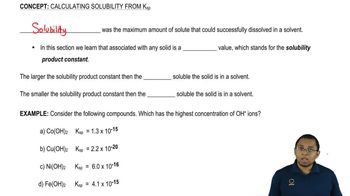01:30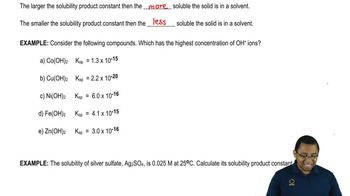01:5905:51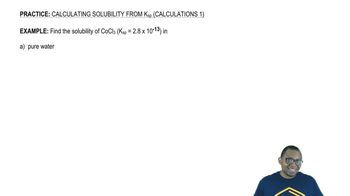05:21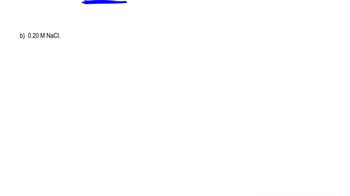04:11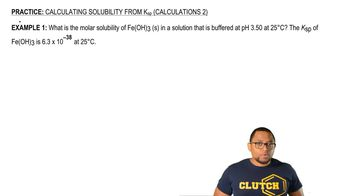04:5005:15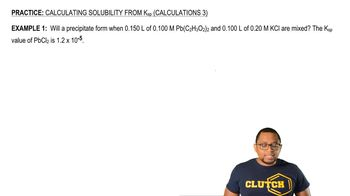07:1606:0503:47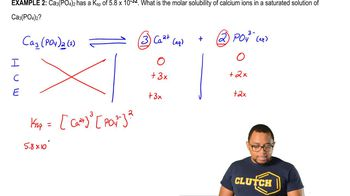04:1003:44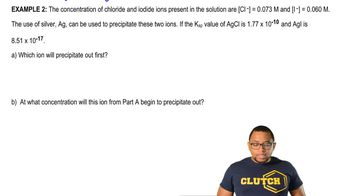03:36